#### 期刊菜单

Analysis of Friction Effect on Recycled Concrete Ends Using Base Force Element Method
DOI: 10.12677/IJM.2021.104020, PDF, HTML, XML, 下载: 190  浏览: 363  国家自然科学基金支持

Abstract: In this paper, a two-dimensional random aggregate mesoscopic model of recycled concrete is de-veloped using the base force element method, which employs Delaunay triangular dissection aligned with the interface, and the constitutive relation of multi-line damage evolution is used for each phase material in the model, and the Kupfer criterion is applied as the basis for determining damage in the biaxial compression zone. The friction between the recycled concrete specimens and the loading plate was considered using the zero-thickness interface element, and the influence of end friction on the peak strength and failure mode of the recycled concrete specimen was discussed. The numerical results are compared well with experimental results. It is proven that the model established in this paper can simulate the macroscopic mechanical behavior of recycled concrete specimens. In addition, the numerical results show that: End friction significantly affects the peak strength and damage mode of recycled concrete, and the degree of influence increases as the aspect ratio decreases; the influence range of end friction is limited, and the farther away from the end, the smaller the influence is.

1. 引言

2. 细观模型简介

2.1. 随机骨料生成及网格划分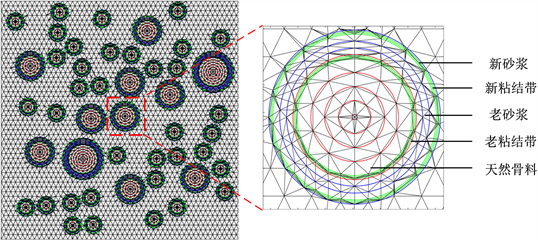Figure 1. Schematic diagram of random aggregate distribution and meshing of recycled aggregate concrete

2.2. 基面力单元法的单元刚度矩阵

${K}^{IJ}=\frac{E}{2A\left(1+\nu \right)}\left[\frac{2\nu }{1-2\nu }{m}^{I}\otimes {m}^{J}+{m}^{IJ}U+{m}^{J}\otimes {m}^{I}\right],\left(I,J=1,2,3\right)$ (1)

${m}^{I}={m}_{\alpha }^{I}{P}_{\alpha }=\frac{1}{2}\left({L}_{IJ}{n}^{IJ}+{L}_{IK}{n}^{IK}\right)$ (2)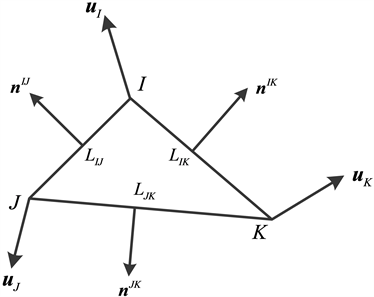Figure 2. A triangular element

2.3. 零厚度界面单元  基本原理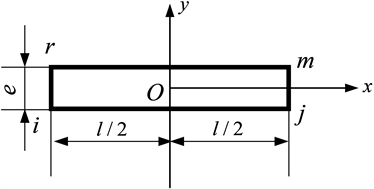Figure 3. A zero-thickness interface element

$\left\{\sigma \right\}=\left\{\begin{array}{l}{\tau }_{s}\\ {\sigma }_{n}\end{array}\right\}=\left[\lambda \right]\left\{\Delta \delta \right\}$ (3)

$\left\{\Delta \delta \right\}=\left\{\begin{array}{c}\Delta u\\ \Delta v\end{array}\right\},\left[\lambda \right]=\left[\begin{array}{cc}{\lambda }_{s}& 0\\ 0& {\lambda }_{n}\end{array}\right]$ (4)

${\left[k\right]}^{e}=\frac{l}{6}\left[\begin{array}{cccccccc}2{\lambda }_{s}& 0& {\lambda }_{s}& 0& -{\lambda }_{s}& 0& -2{\lambda }_{s}& 0\\ 0& 2{\lambda }_{n}& 0& {\lambda }_{n}& 0& -{\lambda }_{n}& 0& -2{\lambda }_{n}\\ {\lambda }_{s}& 0& 2{\lambda }_{s}& 0& -2{\lambda }_{s}& 0& -{\lambda }_{s}& 0\\ 0& {\lambda }_{n}& 0& 2{\lambda }_{n}& 0& -2{\lambda }_{n}& 0& -{\lambda }_{n}\\ -{\lambda }_{s}& 0& -2{\lambda }_{s}& 0& 2{\lambda }_{s}& 0& {\lambda }_{s}& 0\\ 0& -{\lambda }_{n}& 0& -2{\lambda }_{n}& 0& 2{\lambda }_{n}& 0& {\lambda }_{n}\\ -2{\lambda }_{s}& 0& -{\lambda }_{s}& 0& {\lambda }_{s}& 0& 2{\lambda }_{s}& 0\\ 0& -2{\lambda }_{n}& 0& -{\lambda }_{n}& 0& {\lambda }_{n}& 0& 2{\lambda }_{n}\end{array}\right]$ (5)

2.4. 损伤本构模型和强度准则

$\sigma ={E}_{0}\left(1-D\right)\epsilon$ (6)

$E={E}_{0}\left(1-D\right)$ (7)

${D}_{t}=\left\{\begin{array}{ccc}0& & \epsilon \le {\epsilon }_{t0}\\ 1-\frac{{\epsilon }_{t0}}{\epsilon }+\frac{\epsilon -{\epsilon }_{t0}}{{\eta }_{t}{\epsilon }_{t0}-{\epsilon }_{t0}}\frac{{\epsilon }_{t0}}{\epsilon }\left(1-\alpha \right)& & {\epsilon }_{t0}<\epsilon \le {\eta }_{t}{\epsilon }_{t0}\\ 1-\frac{\alpha }{{\xi }_{t}-{\eta }_{t}}\frac{\epsilon -{\eta }_{t}{\epsilon }_{t0}}{\epsilon }+\frac{\alpha {\epsilon }_{t0}}{\epsilon }& & {\eta }_{t}{\epsilon }_{t0}<\epsilon \le {\xi }_{t}{\epsilon }_{t0}\\ 1& & \epsilon >{\xi }_{t}{\epsilon }_{t0}\end{array}$ (8)

${D}_{c}=\left\{\begin{array}{ccc}1-\frac{\beta }{\lambda }& & \epsilon \le \lambda {\epsilon }_{c0}\\ 1-\frac{1-\beta }{1-\lambda }\frac{\epsilon -\lambda {\epsilon }_{co}}{\epsilon }-\beta \frac{{\epsilon }_{co}}{\epsilon }& & \lambda {\epsilon }_{c0}<\epsilon \le {\epsilon }_{c0}\\ 1-\frac{1-\gamma }{1-{\eta }_{c}}\frac{\epsilon -{\epsilon }_{co}}{\epsilon }-\frac{{\epsilon }_{co}}{\epsilon }& & {\epsilon }_{c0}<\epsilon \le {\eta }_{c}{\epsilon }_{c0}\\ 1-\frac{\gamma {\epsilon }_{c0}}{\epsilon }& & {\eta }_{c}{\epsilon }_{c0}<\epsilon \le {\xi }_{c}{\epsilon }_{c0}\\ 1& & \epsilon >{\xi }_{c}{\epsilon }_{c0}\end{array}$ (9)Table 1. Mechanical parameters of each phase material of recycled concrete

${\sigma }_{c}=\frac{1+3.65\alpha }{{\left(1+\alpha \right)}^{2}}{f}_{c}$ (10)

3. 模拟方案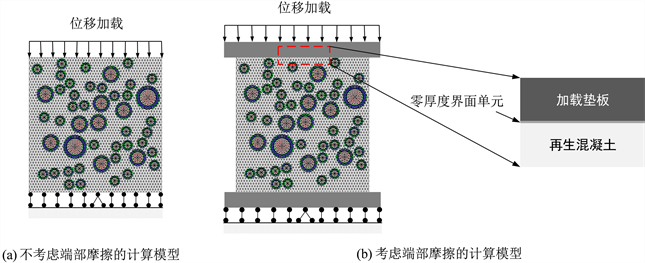Figure 4. Numerical calculation model

4. 计算结果与分析

4.1. 端部摩擦对再生混凝土试件峰值强度的影响Table 2. Peak strength of recycled concrete specimenFigure 5. Peak strength of specimens with different slenderness under different end conditionsTable 3. Comparison between the simulation results and test result

4.2. 端部摩擦对再生混凝土试件破坏模式的影响

4.3. 结果分析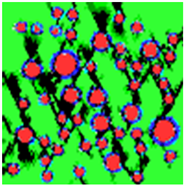(a) 100 mm × 100 mm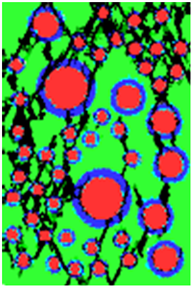(b) 100 mm × 150 mm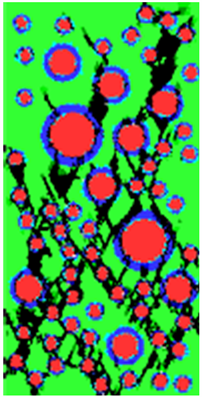(c) 100 mm × 200 mm

Figure 6. The final failure mode of the specimens without considering the end friction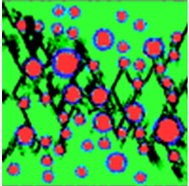(a) 100 mm × 100 mm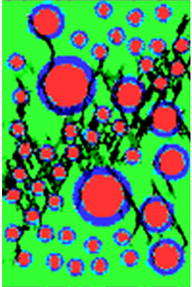(b) 100 mm × 150 mm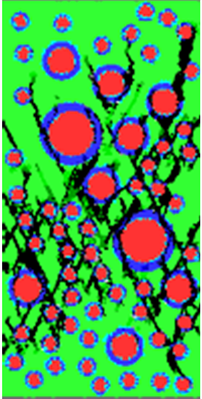(c) 100 mm × 200 mm

Figure 7. The final failure mode of the specimens considering the end friction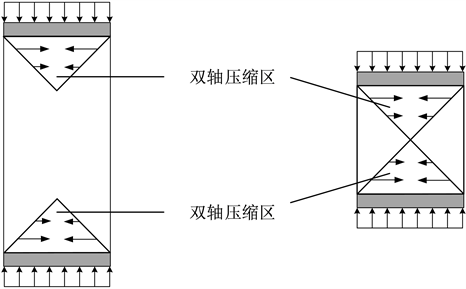Figure 8. Biaxial compression zones of specimens

5. 结论

1) 在基面力单元法中引入零厚度界面单元可较好的用于模拟再生混凝土试件与垫板之间的摩擦，且模拟结果与试验结果一致，证明了本文模型的可靠性。

2) 考虑试件与加载垫板之间的端部摩擦时，再生混凝土试件的高宽比对峰值强度的影响较不考虑端部摩擦时更明显。

3) 端部摩擦会显著影响再生混凝土试件的峰值强度和破坏模式，并且随着高宽比减小，影响程度增大。

  尤明庆, 苏承东. 大理岩试样的长度对单轴压缩试验的影响[J]. 岩石力学与工程学报, 2004, 23(22): 3754-3760.  梁正召, 唐春安, 张娟霞, 等. 岩石三维破坏数值模型及形状效应的模拟研究[J]. 岩土力学, 2007, 28(4): 699-704.  Indelicato, F. and Paggi, M. (2008) Specimen Shape and the Problem of Contact in the Assessment of Concrete Compressive Strength. Materials and Structures, 41, 431-441. https://doi.org/10.1617/s11527-007-9256-7  陈健云, 刘智光. 混凝土试样单轴压缩端面效应及破坏数值模拟[J]. 大连理工大学学报, 2013, 53(1): 90-96.  康政, 唐欣薇, 秦川, 等. 基于细观离散元的混凝土端部效应分析[J]. 哈尔滨工业大学学报, 2013, 45(12): 94-98.  金浏, 韩亚强, 丁子星, 等. 端部摩擦约束对混凝土单轴动态压缩强度影响分析[J]. 振动与冲击, 2016, 35(11): 12-19+33.  唐世斌, 唐春安, 梁正召. 接触面损伤演化过程的数值模型[J]. 计算力学学报, 2011, 28(1): 146-151.  Goodman, R.E., Taylor, R.L. and Brekke, T.L. (1968) A Model for the Mechanics of Jointed Rock. Journal of the Soil Mechanics and Foundations Division, 94, 637-659. https://doi.org/10.1061/JSFEAQ.0001133  Walraven, J.C. and Reinhardt, H.W. (1981) Concrete Mechanics. Part A: Theory and Experiments on the Mechanical Behavior of Cracks in Plain and Reinforced Concrete Subjected to Shear Loading. Nasa Sti/recon Technical Report N, 82, Article No. 25417.  Gao, Y. (2003) A New Description of the Stress State at a Point with Applications. Archive of Applied Mechanics, 73, 171-183. https://doi.org/10.1007/s00419-003-0278-5  Peng, Y. and Liu, Y. (2019) Advances in the Base Force Element Method. Springer, Singapore. https://doi.org/10.1007/978-981-13-5776-3  Peng, Y., Dong, Z., Peng, B. and Liu, Y. (2011) Base Force Element Method (BFEM) on Potential Energy Principle for Elasticity Problems. International Journal of Mechanics and Materials in Design, 7, 245-251. https://doi.org/10.1007/s10999-011-9162-6  朱伯芳. 有限单元法原理与应用[M]. 北京: 水利水电出版社, 1979.  Lemaitre, J. and Chaboche, J.L. (1994). Mechanics of Solid Materials. Cambridge University Press, Cambridge.  彭一江, 应黎坪. 再生混凝土细观分析方法[M]. 北京: 科学出版社, 2018.  刘琼. 再生混凝土破坏机理的试验研究和格构数值模拟[D]: [博士学位论文]. 上海: 同济大学, 2010.  肖建庄, 杜江涛, 刘琼. 基于格构模型再生混凝土单轴受压数值模拟[J]. 建筑材料学报, 2009, 12(5): 511-514+518.  Xiao, J., Li, W., Corr, D.J. and Shah, S.P. (2013) Simulation Study on the Stress Distribution in Modeled Recycled Aggregate Concrete under Uniaxial Compression. Journal of Materials in Civil Engineering, 25, 504-518. https://doi.org/10.1061/(ASCE)MT.1943-5533.0000598  Peng, Y., Chen, X., Ying, L. and Kamel, M.M. (2019) Research on Softening Curve of Recycled Concrete Using Base Force Element Method in Meso-Level. Engineering Computations, 36, 2414-2429. https://doi.org/10.1108/EC-11-2018-0510  Kupfer, H., Hilsdorf, H.K. and Rusch, H. (1969) Behavior of Concrete under Biaxial Stresses. Journal Proceedings, 66, 656-666. https://doi.org/10.14359/7388  李守德, 俞洪良. Goodman接触面单元的修正与探讨[J]. 岩石力学与工程学报, 2004, 23(15): 2628-2631.  梁正召, 吴宪锴, 唐世斌. 基于各向异性界面单元的岩石端部效应数值模拟[J]. 应用基础与工程科学学报, 2018, 26(3): 526-537.  Van Vliet, M.R.A. and Van Mier, J.G.M. (1995) Softening Behaviour of Concrete under Uniaxial Compression. Fracture Mechanics of Concrete Structures, 1, 383.  胡雄志. 复杂应力状态下再生混凝土本构关系研究[D]: [硕士学位论文]. 北京: 北方工业大学, 2017.